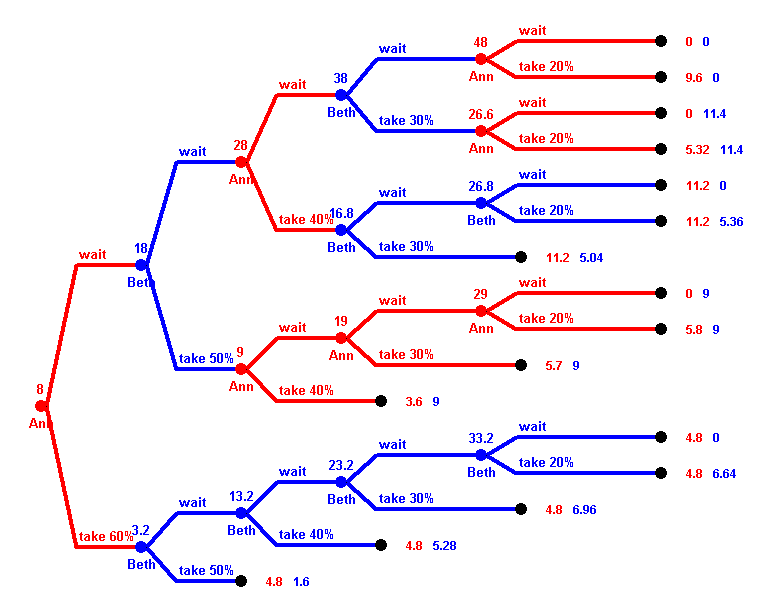# Test 2

#### Question 1:

Two players, Ann and Beth, play the following game. At the beginning 8 Dollars lie on the desk. There are 5 rounds, where, starting with Ann, the players that have not yet "finished" alternately move. A player who is to move can either take part of the money on the desk, or wait. A player can only take money once---a player who takes some money has "finished" and cannot move anymore. If one player has already finished, the other one moves every round. In the first round, the player who is to move can take 60% of the money, in the second round 50%, in the third round 40%, and so on. But in rounds where no money is taken, where the player waits, the money on the desk increases by \$10.So, on the one hand, the money increases if the players wait, on the other hand the percentage they are allowed to take decreases over time, and moreover the money can decreases when the other player takes some. So the trick is to find the right time to take money.

The game tree is shown above. At every vertex the amount of money on the desk at that time is written above the vertex, and the name of the player to move below. At the terminal vertices, the payoffs for Ann and beth are given.

Use backwards induction to answer the following questions:

1. (1 point) What is Ann's first move, when playing optimally?
2. (1 point) What is Beth's move in round 2 when she plays optimally? Does it depend on Ann's previous move? If yes, how?
3. (1 point) How much money does Ann and Beth get when both play optimally?
4. (1 point) If Ann plays optimally but Beth not necessarily, how much money can Ann win? Is there some minimum she still can guarantee?

#### Question 2:

a) (1 point) What is the probability of drawing a king from a deck of 52 cards?

b) (1 point) What is the probability of drawing a red card or a king from a deck of 52 cards?

#### Question 3:

(1 point) An experiment has three equally likely outcomes. The payoffs for them are \$2,\$3, and -\$1. What is the expected payoff of the experiment?

#### Question 4:

(1 points) You have four coins in your pocket: one Euro coins, two 50c, and one 10c. You choose on randomly. What is the expected value?

(1 points) In the same situation as above, what is the expected value if you pick two coins randomly?